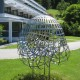#### [SOLVED] Reference needed: Isomorphism on pi_1 and homology gives weak equivalence

Let $f : X \to Y$ be a map between a connected space $X$ and a space $Y$. If $\pi(f) : \pi_1(X) \to \pi_1(Y)$ is an isomorphism, and $H_n(f) : H_n(X, G) \to H_n(Y, G)$ is an isomorphism for all $n \ge 1$ and for any local system of coefficients $G$, then $X$ is weakly equivalent to $Y$. Does anyone have a reference (or proof) for this?#### @John Klein 2011-02-13 04:41:29

The proof in the simply connected case is well-known and is a consequence of the relative Hurewicz theorem. See Corollary 1, page 79 of Mosher and Tangora's book, Cohomology Operations and Applications in Homotopy Theory for this case.

If $X$ and $Y$ aren't 1-connected, then $f$ lifts to a map of universal covers $\tilde f: \tilde X \to \tilde Y$ and your assumption about local coefficients implies that $\tilde f$ is a homology isomorphism. We can therefore apply the previous paragraph to show that $\tilde f$ is a weak equivalence. This implies that $f$ is since $f$ is a $\pi_1$-isomorphism.#### @Paul 2011-02-13 04:47:10

So what's a simple counterexample to the problem as stated?#### @John Klein 2011-02-13 04:58:21

@Paul: A high dimensional knot knot complement with $\pi_1 = \Bbb Z$ will give a counterexample. Let $S^n \subset S^{n+2}$ be a $n$-knotted sphere and $X$ its complement ($n > 2$). Assume $\pi_1(X)$ is $\Bbb Z$ (it is not hard to find such knots). Let $f:X\to S^1$ be a cohomology generator. Then $f$ is a $\pi_1$-isomorphism and a homology isomorphism. But $f$ isn't a weak equivalence, since that would imply the knot is trivial (by Levine).#### @Dan Ramras 2011-02-13 00:45:36

You need to assume either that the spaces involved are simple (I believe Emmanuel Dror-Farjoun generalized that to nilpotent), or that the map f induces an isomorphism in homology with local coefficients. There is an exercise in Hatcher's book that discuss this, in Section 4.2 (Ex. 12). You should also look at Peter May's beautiful paper The Dual Whitehead Theorems, in Peter Hilton's birthday conference proceedings.#### @Joris Weimar 2011-02-13 01:38:15

yes, sorry, i forgot the local coefficients condition. thank you!#### @Mariano Suárez-Álvarez 2011-02-13 00:32:22

Switzer proves in his Algebraic Topology as theorem 10.28 that statement for $1$-connected spaces CW-complexes.